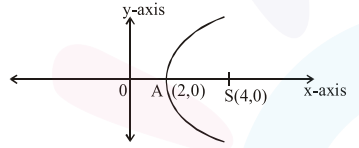# Axis of a parabola lies along x-axis.`
Question:

Axis of a parabola lies along $x$-axis. If its vertex and focus are at distances 2 and 4 respectively from the origin, on the positive $x$-axis then which of the following points does not lie on it ?

1. $(4,-4)$

2. $(5,2 \sqrt{6})$

3. $(8,6)$

4. $6,4 \sqrt{2}$

Correct Option: 3,

Solution:equation of parabola is

$y^{2}=8(x-2)$

$(8,6)$ does not lie on parabola.